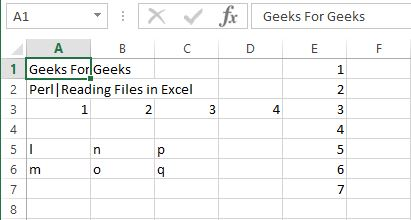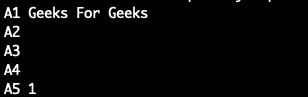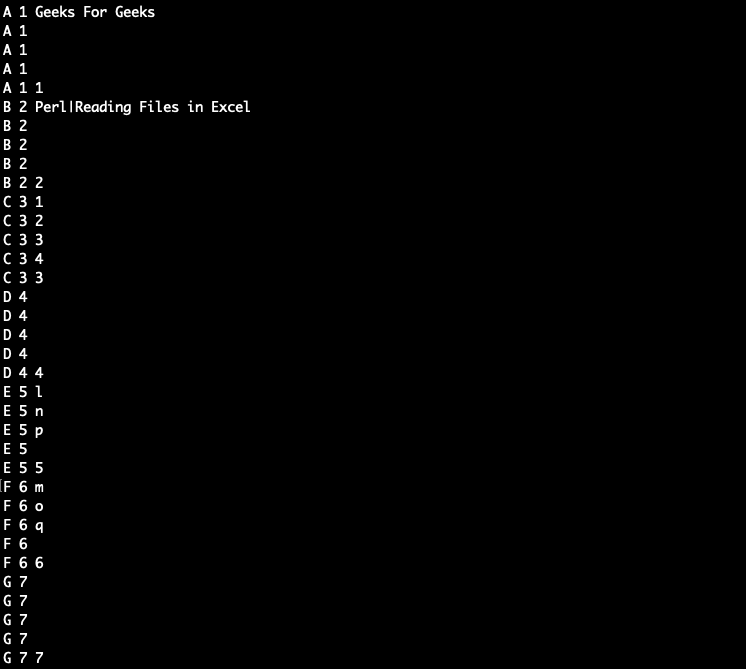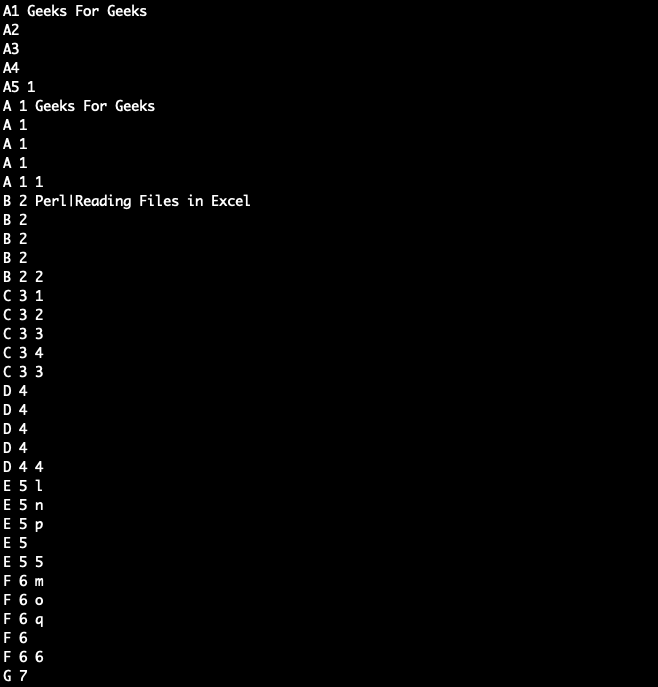Related Articles
Perl | Reading Excel Files
• Last Updated : 11 Jul, 2019

Excel sheets are one of the most commonly used methods for maintaining office records, especially to work on applications where non-developers and even managers can provide input to the systems in batches.

However, the issue is to read the content from a file created by Microsoft Excel using Perl.

Few modules for reading from Excel files are offered by CPAN. There is `Spreadsheet::Read` that will be able to handle all types of spreadsheets. There are other low-level libraries reading files by different versions of Excel:

• Spreadsheet::ParseExcel Excel 95-2003 files,
• Spreadsheet::ParseXLSX Excel 2007 Open XML XLSX

### Creation of an Excel File

Excel files can be created with the use of Perl by the help of an inbuilt module Excel::Writer::XLSX which is used to create Excel files.
Further, `write()` function is used to add content to the excel file.
Example:

 `#!/usr/bin/perl``use` `Excel::Writer::XLSX;``my` `\$Excel_book1`  `= Excel::Writer::XLSX->new(``'new_excel.xlsx'` `);``my` `\$Excel_sheet1` `= ``\$Excel_book1``->add_worksheet();``my` `@data_row` `= (1, 2, 3, 4);``my` `@table_data` `= (``    ``[``"l"``, ``"m"``],``    ``[``"n"``, ``"o"``],``    ``[``"p"``, ``"q"``],``);``my` `@data_column` `= (1, 2, 3, 4, 5, 6, 7);`` ` `# Using write() to write values in sheet``\$Excel_sheet1``->``write``( ``"A1"``, ``"Geeks For Geeks"` `);``\$Excel_sheet1``->``write``( ``"A2"``, ``"Perl|Reading Files in Excel"` `);``\$Excel_sheet1``->``write``( ``"A3"``, \``@data_row` `);``\$Excel_sheet1``->``write``( 4, 0, \``@table_data` `);``\$Excel_sheet1``->``write``( 0, 4, [ \``@data_column` `] );``\$Excel_book1``->``close``;`### Reading from a Excel File

Reading of an Excel File in Perl is done by using `Spreadsheet::Read` module in a Perl script. This module exports a number of function that you either import or use in your Perl code script. `ReadData()` function is used to read from an excel file.
The `ReadData()` function accepts a filename which in this case is an Excel file, but it also accepts various other file types. Based on the file-extension, it will load the appropriate back-end module, then parses the file. It creates an array reference which represents the whole file:
Example:

 `use` `5.016;``use` `Spreadsheet::Read ``qw(ReadData)``;``my` `\$book_data` `= ReadData (‘new_excel.xlsx');``say` `'A2: '` `. ``\$book_data``->{A2};`

In the above code, the first element of the array which has been returned contains general information about the file. The remaining elements represent the other sheets in the file. In other words, \$book_data-> represents the first sheet of the ‘new_excel.xlsx’. This can be used to access the content of the cells as it is a hash reference. \$book_data->{A2} returns a hash reference for A2 element

Output:

`A2: Perl|Reading Files in Excel`

### Fetching Rows of an Excel File

The arguments of the function of `Spreadsheet::Read` are a sheet, and the number of the rows to be fetched. The return type is an array with the values of the rows passed in the argument.
The following program demonstrates how to read the first row of the first sheet, and then displays the content in each field of the row.

 `my` `@rowsingle` `= Spreadsheet::Read::row(``\$book_data``->, 1);``for` `my` `\$i` `(0 .. ``\$#rowsingle``)``{``    ``say` `'A'` `. (``\$i` `+ 1) . ``' '` `. ``              ``(``\$rowsingle``[``\$i``] // ``''``);``}`

Output:### Fetching File content

Fetching a single row is not nearly enough. We need to fetch all the rows for efficient programming. We accomplish this using the `rows()` function. This function takes a sheet as an argument. It returns an array of elements or array of references as a matrix(2-D array). Each element in the matrix represents a row in the spreadsheet.
The script to fetch all rows is as follows:

 `my` `@rowsmulti` `= Spreadsheet::Read::rows(``\$book_data``->);``foreach` `my` `\$m` `(1 .. ``scalar` `@rowsmulti``) ``{``    ``foreach` `my` `\$n` `(1 .. ``scalar` `@{``\$rowsmulti``[``\$m` `- 1]}) ``    ``{``        ``say` `chr``(64 + ``\$m``) . ``" \$m "` `.``               ``(``\$rowsmulti``[``\$m` `- 1][``\$n` `- 1] // ``''``);``    ``}``}`

Output:Putting it all together
Following Perl script illustrates the use of all the above explained Features of Reading an Excel File in Perl:

 `#!/usr/bin/perl``use` `strict;``use` `warnings;``use` `5.010;`` ` `use` `Spreadsheet::Read ``qw(ReadData)``;`` ` `my` `\$bookdata` `= ReadData(``'simplecreate.xlsx'``);`` ` `say` `'A1: '` `. ``\$bookdata``->{A1}; `` ` `# Fetching a single row``my` `@rowsingle` `= Spreadsheet::Read::row(``\$bookdata``->, 1);``for` `my` `\$i` `(0 .. ``\$#row``) ``{``    ``say` `'A'` `. (``\$i` `+ 1) . ``' '` `. ``              ``(``\$rowsingle``[``\$i``] // ``''``);``}`` ` `# Fetching all file content``my` `@rowsmulti` `= Spreadsheet::Read::rows(``\$bookdata``->);``foreach` `my` `\$i` `(1 .. ``scalar` `@rowsmulti``) ``{``    ``foreach` `my` `\$j` `(1 .. ``scalar` `@{``\$rows``[``\$i``-1]}) ``    ``{``        ``say` `chr``(64 + ``\$i``) . ``" \$j "` `. ``               ``(``\$rows``[``\$i` `- 1][``\$j` `- 1] // ``''``);``    ``}``}`

OutPut:Attention reader! Don’t stop learning now. Get hold of all the important DSA concepts with the DSA Self Paced Course at a student-friendly price and become industry ready.

My Personal Notes arrow_drop_up